```EXPERIMENTAL: AN INVESTIGATION TO DETERMINE THE RELATIONSHIP BETWEEN
TEMPERATURE RISE AND TIME WHEN A SURFACE IS HEATED BY A RADIANT HEAT
SOURCE
Introduction
Extensive studies have shown that the amount of radiant energy absorbed
by a surface is affected by a number of independent variables; these
include the total and type of energy given out from the heat source,
the distance of the surface from the source, the colour of the surface,
and its lustre (i.e., whether the surface is shiny or dull).
Preliminary studies have shown that the temperature rise (DT) of a
dark-coloured surface, over a period of 300 seconds, is related to time
(t) by the following linear relationship: ```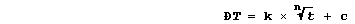```where 'k' is a proportionality constant, 'n' is an index, and 'c' is a
constant. However, the 'best' value for 'n' remains to be established;
in principle, a combination of experimental and graphical methods can
be used to obtain this value ... ```
 ```Using raw data collected by the experimental method described, the focus of your investigation is determine whether there is evidence to support one of the following hypotheses. Hypoth. 1: The temperature rise of a surface is in linear proportion to the time exposed to radiant heat energy; i.e.,```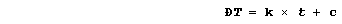```Hypoth. 2: The temperature rise of a surface is in linear proportion to the square root of the time exposed to radiant heat energy; i.e.,```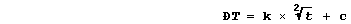```Hypoth. 3: The temperature rise of a surface is in linear proportion to the cube root of the time exposed to radiant heat energy; i.e.,```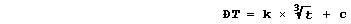```Hypoth. 4: The temperature rise of a surface is in linear proportion to the fourth root of the time exposed to radiant heat energy; i.e.,```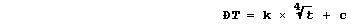```Method
1.  Using a reference thermometer, measure room temperature.
2.  Carefully clamp a 0-50°C black-bulb thermometer at a measured
height from the bench.
3.  Place a 100 W light bulb 0.060 m away from this thermometer; this
distance is measured, using a pair of dividers, from the light bulb
side-surface to the bulb of the thermometer.
4.  Measure the starting temperature of the black-bulb thermometer to
the nearest 0.1°C.
5.  Switch on the 100 W light bulb, and record the temperature every
60 seconds for 300 seconds.
6.  Repeat step 5 twice more, using the same starting temperature;
thus, cool the thermometer to below this value, clamp it in position,
and then allow it to reach the required starting temperature.
7.  Finally, to determine whether the temperature gradient has changed,
use the reference thermometer to re-measure room temperature.
Table of Results and Calculations
Constants:_____________________________________________________________
_______________________________________________________________________
_______________________________________________________________________
_______________________________________________________________________```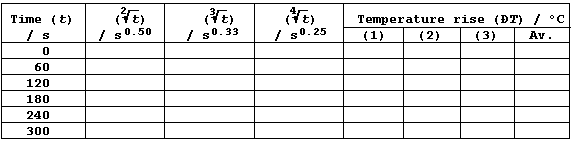```[The average value for DT is obtained either from all three results, if
they are acceptably close together, or from the two closest results.]
Notes
Complete this investigation, as follows.  First, construct a graph for
each form of the independent variable (with a gradient if appropriate);
you will need to truncate the horizontal axis when the variable is the
square-root, cube-root, or fourth-root of time (t).  Second, write a
critical evaluation. And third, construct a precisely worded conclusion
for each form of the independent variable.
Dr. R. Peters                  Next                      Contents' List```